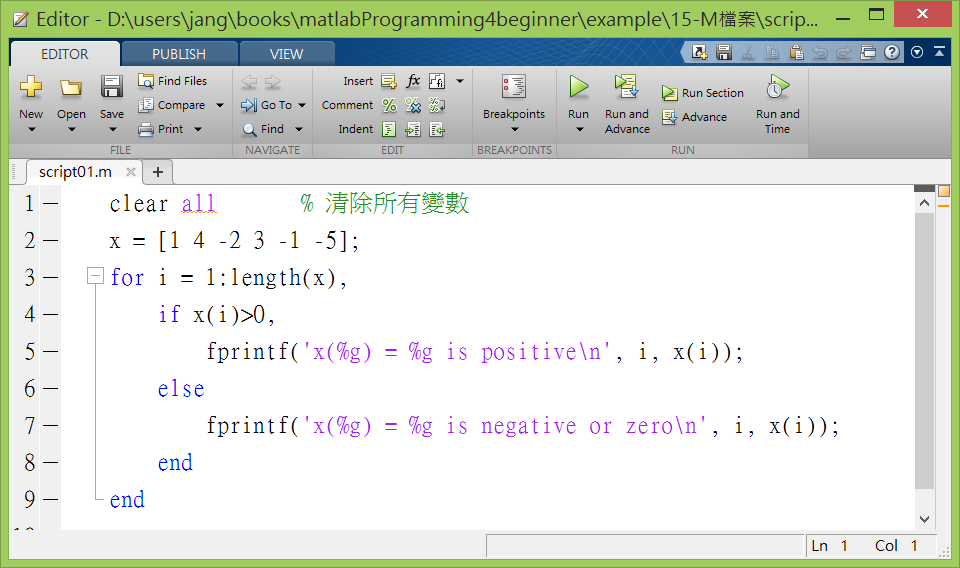15-1 嚙踝蕭嚙踝蕭嚙踝蕭

>> cd ’d:\matlabBook\MATLAB程式設計：入門篇\15-M檔案’ >> type script01.m clear all % 清除所有變數 x = [1 4 -2 3 -1 -5]; for i = 1:length(x), if x(i)>0, fprintf('x(%g) = %g is positive\n', i, x(i)); else fprintf('x(%g) = %g is negative or zero\n', i, x(i)); end end

>> script01 x(1) = 1 is positive x(2) = 4 is positive x(3) = -2 is negative or zero x(4) = 3 is positive x(5) = -1 is negative or zero x(6) = -5 is negative or zero

>> whos Name Size Bytes Class i 1x1 8 double array x 1x6 48 double array Grand total is 7 elements using 56 bytes

Hint

M 檔案是一個文字檔，所以您可以用各種文字編輯器去修改他，但記得在儲存檔案時，必需以文字模式儲存。此外，MATLAB 在 Windows 及 Mac 的平台上，亦提供了一個內建的「M 檔案編輯器」（M-File Editor），您可以點選 MATLAB 指令視窗的 file/open 下拉式選單，來開啟 M 檔案編輯器，另一種更快的方法，則是在 MATLAB 指令視窗只接鍵入「edit filename.m」 或是「open filename.m」。例如 ，欲開啟 Script01.m，可輸入如下：

>> edit script01.mM 檔案編輯器會以不同的顏色來顯示註解、關鍵字、字串、及一般程式碼，在使用上相當方便。

Hint

MATLAB程式設計：入門篇